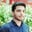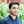Trusted answers to developer questions
Trusted Answers to Developer Questions

Related Tags

r
communitycreator

# What is the mode() method in R?Salman Yousaf

Grokking Modern System Design Interview for Engineers & Managers

Ace your System Design Interview and take your career to the next level. Learn to handle the design of applications like Netflix, Quora, Facebook, Uber, and many more in a 45-min interview. Learn the RESHADED framework for architecting web-scale applications by determining requirements, constraints, and assumptions before diving into a step-by-step design process.

The mode() method in R extracts the most frequently occurring value(s) in a vector x or data frame.

### Syntax

The syntax of the mode() function to calculate the most frequent value(s) in the vector x is as follows:

mode(x, na.rm = FALSE)


### Parameters

• x: A numerical or character type non-empty vector.
• na.rm: A boolean field. na.rm can be set to TRUE to remove NA(Not Available) values before mean calculation; the default value is False.

### Return value

• Number
• Character
• Vector
• NA

The return value of mode() depends on the type of x, i.e., it can return a number or character. If multiple numbers occur most frequently, then mode() will return a vector.

### Code

We define Mode in line 5. The function takes vector x with the default value of na.rm = FALSE. In line 16, it computes the mode of vector x = (1,2,3,4,5,6,2,5,6,2) and returns 2.

# Create a vector.
x <- c(1,2,3,4,5,6,2,5,6,2)

# Own Mode method to calculate mode
Mode <- function(x, na.rm = FALSE) {
# it takes two areguments x, and na.rm
if(na.rm){ #if na.rm is false it means no need to remove NA values
x = x[!is.na(x)]
}

valx <- unique(x)
return(valx[which.max(tabulate(match(x, valx)))])
}

# Evaluate mode of numbers
mode_value <-  Mode(x)
typeof(mode_value)
# Printing results
cat("Mode is:", mode_value)

In the example below, x = (6,14,6,14.2,18,NA,2,63,-21,9,-5,-4). Now, the vector has an NA value as well. The Mode() method takes na.rm = TRUE as an argument and ignores the NA value to compute the mode in line 16.

# Create a vector.
x <- c(6,14,6,14.2,18,NA,2,63,-21,9,-5,-4)

# Own Mode method to calculate mode
Mode <- function(x, na.rm = FALSE) {
# it takes two areguments x, and na.rm
if(na.rm){ #if na.rm is false it means no need to remove NA values
x = x[!is.na(x)]
}

valx <- unique(x)
return(valx[which.max(tabulate(match(x, valx)))])
}

# Find mode dropping NA values.
mode_value <-  Mode(x,na.rm = TRUE)
cat("\nAfter removing, Mode is: ", mode_value)

RELATED TAGS

r
communitycreator

CONTRIBUTORSalman Yousaf

Grokking Modern System Design Interview for Engineers & Managers

Ace your System Design Interview and take your career to the next level. Learn to handle the design of applications like Netflix, Quora, Facebook, Uber, and many more in a 45-min interview. Learn the RESHADED framework for architecting web-scale applications by determining requirements, constraints, and assumptions before diving into a step-by-step design process.

Keep Exploring

Learn in-demand tech skills in half the time